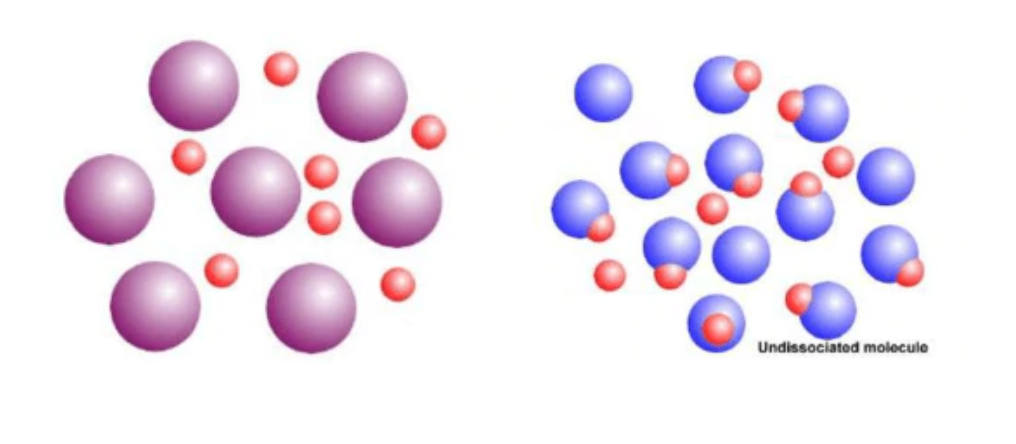# Calculating pH and pOH

This is part of the HSC Chemistry course under the topic Using Brønsted-Lowry Theory.

### HSC Chemistry Syllabus

• Calculate pH, pOH, hydrogen ion concentration ([H+]) and hydroxide ion concentration ([OH]) for a range of solutions (ACSCH102)

### Acidity

• Acids by various definitions are hydrogen ion (H+) producers (Arrhenius Theory) or proton donors (Brønsted-Lowry Theory)
• Not all hydrogen containing molecules are acids. Molecules are only considered to be acids if their hydrogen ions can be ionised.• The acidity of a solution is measured by pH which stands for the potential of hydrogen. It is calculated using the formula

$$pH = -log_{10}[H^+]$$

Or the hydrogen ion concentration can be calculated using the formula

$$[H^+] = 10^{-pH}$$

• pH is measured on a logarithmic scale which means that a change in value of 1 corresponds to a ten-fold change

pH = 1 corresponds to [H+] of 0.1 mol L–1

pH = 2 corresponds to [H+] of 0.01 mol L–1

• Notice that a smaller pH corresponds to an increasing concentration of hydrogen ions. This means a smaller pH will mean a more acidic solution

### Basicity

• The basicity of a solution can be measured with pOH. Similar to pH, a change in value of 1 corresponds to a tenfold change in [OH].

$$pOH = -log_{10}[OH^-]$$

Or the hydroxide concentration can be calculated using the formula

$$[OH^-] = 10^{-pOH}$$

### Calculation of pH and pOH

• It is important to recognise that significant figures for hydrogen ion and hydroxide ion concentration, are expressed as decimal places when calculating pH and pOH

Example: Calculate the pH for a solution with hydrogen ion concentration of 0.0010 mol L–1

• Looking at our example the hydrogen ion concentration 0.0010 is given as 2 significant figures. This means that we will have to express our pH to 2 decimal places

To calculate pH, use the formula

$$pH = -log_{10}[H^+]$$

$$pH = -log_{10}[0.0010] = 3$$

However remember that we must express our answer to 2 decimal places

$$\therefore pH = 3.00$$

Example: Calculate the concentration of hydrogen ions in a solution of pH 4.35

• This time we are going from pH to a hydrogen ion concentration. The pH has 2 decimal places so we need to give our answer in 2 significant figures.

To calculate the hydrogen ion concentration, we use the formula

$$[H^+] = 10^{-pH}$$

$$[H^+] = 10^{-4.35} = 4.466735922 \times 10^{-5} \hspace{0.1cm} mol \hspace{0.1cm} L^{-1}$$

However remember that we must express our answer to 2 significant figures

$$\therefore [H^+] = 4.5 \times 10^{-5} \hspace{0.1cm} mol \hspace{0.1cm} L^{-1}$$

### Relationship between pH and pOH

• Self-ionisation of water is represented by the reaction:

$$2H_2O(l) \rightleftharpoons H_3O^+(aq) + OH^-$$

• The equilibrium constant, Kw, of this reaction = 10–14 at 25 ºC. This is extremely small which indicates that the equilibrium lies on the far-left side of the reaction, resulting in very small amounts of H3O+ and OH equilibrium.

$$\boldsymbol{K_w} = [H_3O^+_{(aq)}][OH^-_{(aq)}] = 10^{-14}$$

• pH and pOH can be calculated using the following equation:
Derivation (do not memorise):

$$\boldsymbol{log}([H_3O^+_{(aq)}][OH^-_{(aq)}]) = log(10^{-14})$$

$$\boldsymbol{log}[H_3O^+_{(aq)}] + \boldsymbol{log}[OH^-_{(aq)}] = -14$$

$$\boldsymbol{-log}[H_3O^+_{(aq)}] - \boldsymbol{log}[OH^-_{(aq)}] = 14$$

$$pH + pOH = 14$$

### Effect of Dilution on pH, Degree of Ionisation and Ka

• Adding water to an acid solution reduces the concentration of all chemical species in the solution. As [H+] decreases, pH increases.

• However, Le Chatelier’s principle also applies when dilution occurs as water (reactant) is added to the system. This will shift the equilibrium to the right (product) side to form more H+/H3O+ ions in order to increase the concentration of ions in the system. As a result, the degree of dissociation increases upon dilution of acid.

• Besides Ka, the degree of dissociation or ionisation of an acid is another quantitative measure of its strength. Degree ionisation is the percentage of weak acid (HA) molecules that ionises in solution.

The degree of dissociation is given by the formula:

$$\frac{[H^+]}{initial[HA]} \times 100$$

• Although acid dissociates more upon dilution, the effect of dilution, compared to equilibrium shift, has a much greater effect on [H+]. This means pH always increases upon dilution.

Table: the effect of adding water or acid to a solution of acid on its pH and degree of dissociation

 Action Effect on pH Effect on degree of dissociation Effect on K­a Adding water Increases Increases Unchanged Adding acid Decreases Decreases Unchanged

• Although the addition of water increases the degree of ionisation of an acid, the Ka remains unchanged since the ratio of generic acid [HA] to [H+] and conjugate base [A-] is the same.
• While it may not seem this way, the change in concentration between the different species is proportional to one another

$$[HA] \propto [H^+] \propto [A^-]$$

• The only factor change which affects Ka is a change in temperature